# 15.5: Chemical Reactions of Alcohols. Reactions Involving the O-H Bond

$$\newcommand{\vecs}{\overset { \rightharpoonup} {\mathbf{#1}} }$$ $$\newcommand{\vecd}{\overset{-\!-\!\rightharpoonup}{\vphantom{a}\smash {#1}}}$$$$\newcommand{\id}{\mathrm{id}}$$ $$\newcommand{\Span}{\mathrm{span}}$$ $$\newcommand{\kernel}{\mathrm{null}\,}$$ $$\newcommand{\range}{\mathrm{range}\,}$$ $$\newcommand{\RealPart}{\mathrm{Re}}$$ $$\newcommand{\ImaginaryPart}{\mathrm{Im}}$$ $$\newcommand{\Argument}{\mathrm{Arg}}$$ $$\newcommand{\norm}{\| #1 \|}$$ $$\newcommand{\inner}{\langle #1, #2 \rangle}$$ $$\newcommand{\Span}{\mathrm{span}}$$ $$\newcommand{\id}{\mathrm{id}}$$ $$\newcommand{\Span}{\mathrm{span}}$$ $$\newcommand{\kernel}{\mathrm{null}\,}$$ $$\newcommand{\range}{\mathrm{range}\,}$$ $$\newcommand{\RealPart}{\mathrm{Re}}$$ $$\newcommand{\ImaginaryPart}{\mathrm{Im}}$$ $$\newcommand{\Argument}{\mathrm{Arg}}$$ $$\newcommand{\norm}{\| #1 \|}$$ $$\newcommand{\inner}{\langle #1, #2 \rangle}$$ $$\newcommand{\Span}{\mathrm{span}}$$

## Acidic Properties

Several important chemical reactions of alcohols involve only the oxygen-hydrogen bond and leave the carbon-oxygen bond intact. An important example is salt formation with acids and bases. Alcohols, like water, are both weak bases and weak acids. The acid ionization constant $$\left( K_\text{a} \right)$$ of ethanol is about $$10^{-18}$$, slightly less than that of water. Ethanol can be converted to its conjugate base by the conjugate base of a weaker acid such as ammonia $$\left( K_\text{a} \sim 10^{-35} \right)$$, or hydrogen $$\left( K_\text{a} \sim 10^{-38} \right)$$. It is convenient to employ sodium metal or sodium hydride, which react vigorously but controllably with alcohols: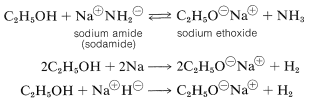The order of acidity of various liquid alcohols generally is water $$>$$ primary $$>$$ secondary |(>\) tertiary $$\ce{ROH}$$. By this we mean that the equilibrium position for the proton-transfer reaction (Equation 15-1) lies more on the side of $$\ce{ROH}$$ and $$\ce{OH}^\ominus$$ as $$\ce{R}$$ is changed from primary to secondary to tertiary; therefore, tert-butyl alcohol is considered less acidic than ethanol:

$\ce{ROH} + \ce{OH}^\ominus \rightleftharpoons \ce{RO}^\ominus + \ce{HOH} \tag{15-1}$

However, in the gas phase the order of acidity is reversed, and the equilibrium position for Equation 15-1 lies increasingly on the side of $$\ce{RO}^\ominus$$ as $$\ce{R}$$ is changed from primary to secondary to tertiary. tert-Butyl alcohol is therefore more acidic than ethanol in the gas phase. This seeming contradiction appears more reasonable when one considers what effect solvation (or the lack of it) has on equilibria expressed by Equation 15-1. In solution, the larger anions of alcohols, known as alkoxide ions, probably are less well solvated than the smaller ions, because fewer solvent molecules can be accommodated around the negatively charged oxygen in the larger ions: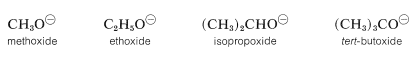Acidity of alcohols therefore decreases as the size of the conjugate base increases. However, “naked” gaseous ions are more stable the larger the associated $$\ce{R}$$ groups, probably because the larger $$\ce{R}$$ groups can stabilize the charge on the oxygen atom better than the smaller $$\ce{R}$$ groups. They do this by polarization of their bonding electrons, and the bigger the group, the more polarizable it is. (Also see Section 11-8A, which deals with the somewhat similar situation encountered with respect to the relative acidities of ethyne and water.)

## Basic Properties

Alcohols are bases similar in strength to water and accept protons from strong acids. An example is the reaction of methanol with hydrogen bromide to give methyloxonium bromide, which is analogous to the formation of hydroxonium bromide with hydrogen bromide and water:Figure 15-4). The melting point reaches a maximum at 50-50 mole percent of each component.Figure 15-4: Melting points of mixtures of methanol and hydrogen bromide

## Nucleophilic Properties - Ether Formation

Alkoxide ion formation is important as a means of generating a strong nucleophile that will readily form $$\ce{C-O}$$ bonds in $$S_\text{N}2$$ reactions. Thus ethanol reacts very slowly with methyl iodide to give methyl ethyl ether, but sodium ethoxide in ethanol solution reacts quite rapidly:In fact, the reaction of alkoxides with alkyl halides or alkyl sulfates is an important general method for the preparation of ethers, and is known as the Williamson synthesis. Complications can occur because the increase of nucleophilicity associated with the conversion of an alcohol to an alkoxide ion always is accompanied by an even greater increase in eliminating power by the $$E2$$ mechanism. The reaction of an alkyl halide with alkoxide then may be one of elimination rather than substitution, depending on the temperature, the structure of the halide, and the alkoxide (Section 8-8). For example, if we wish to prepare isopropyl methyl ether, better yields would be obtained if we were to use methyl iodide and isopropoxide ion rather than isopropyl iodide and methoxide ion because of the prevalence of $$E2$$ elimination with the latter combination:Potassium tert-butoxide is an excellent reagent to achieve $$E2$$ elimination because it is strongly basic and so bulky as to not undergo $$S_\text{N}2$$ reactions readily.

## Nucleophilic Properties. Ester Formation

An ester may be thought of as a carboxylic acid in which the acidic proton has been replaced by some organic group, $$\ce{R}$$,Esters can be prepared from carboxylic acids and alcohols provided an acidic catalyst is present,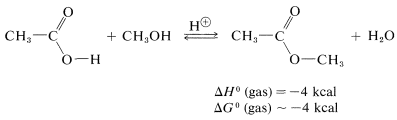or they can be prepared from acyl halides and alcohols or carboxylic anhydrides and alcohols:These reactions generally can be expressed by the equation$$+ \ce{ROH} \rightarrow$$$$+ \ce{HX}$$ which overall is a nucleophilic displacement of the $$\ce{X}$$ group by the nucleophile $$\ce{ROH}$$. However, the mechanism of displacement is quite different from the $$S_\text{N}2$$ displacements of alkyl derivatives, $$\ce{R'X} + \ce{ROH} \rightarrow \ce{R'OR} + \ce{HX}$$, and closely resembles the nucleophilic displacements of activated aryl halides (Section 14-6B) in being an addition-elimination process.

Acyl halides have a rather positive carbonyl carbon because of the polarization of the carbon-oxygen and carbon-halogen bonds. Addition of a nucleophilic group such as the oxygen of an alcohol occurs rather easily.The complex $$1$$ contains both an acidic groupand a basic group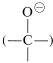, so that a proton shifts from one oxygen to the other to give $$2$$, which then rapidly loses hydrogen chloride by either an $$E1$$- or $$E2$$-type elimination to form the ester.

eliminationA similar but easily reversible reaction occurs between alcohols and carboxylic acids, which is slow in either direction in the absence of a strong mineral acid. The catalytic effect of acids, such as $$\ce{H_2SO_4}$$, $$\ce{HCl}$$, and $$\ce{H_3PO_4}$$ is produced by protonation of the carbonyl oxygen of the carboxylic acid, thereby giving $$3$$. This protonation greatly enhances the affinity of the carbonyl carbon for an electron pair on the oxygen of the alcohol (i.e., $$3 \rightarrow 4$$).

protonation step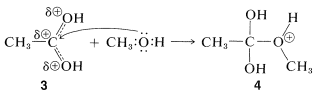Subsequently, a proton is transferred from the $$\ce{OCH_3}$$ to an $$\ce{OH}$$ group of $$4$$ to give $$5$$. This process converts the $$\ce{OH}$$ into a good leaving group $$\left( \ce{H_2O} \right)$$. When $$\ce{H_2O}$$ leaves, the product, $$6$$, is the conjugate acid of the ester. Transfer of a proton from $$6$$ to a base such as $$\ce{H_2O}$$ or $$\ce{HSO_4^-}$$ completes the reaction, giving the neutral ester and regenerating the acid catalyst.

proton transfer and elimination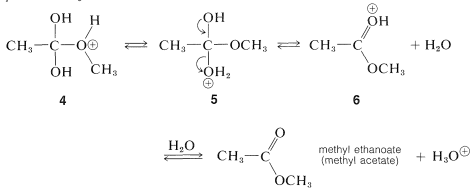Although a small amount of strong acid catalyst is essential in the preparation of esters from acids and alcohols, the amount of acid catalyst added must not be too large. The reason for the “too much of a good thing” behavior of the catalyst can be understood from the basic properties of alcohols (Section 15-4B). If too much acid is present, then too much of the alcohol is converted to the oxonium salt:Clearly, formation of the methyloxonium ion can operate only to reduce the nucleophilic reactivity of methanol toward the carbonyl carbon of the carboxylic acid.

Another practical limitation of esterification reactions is steric hindrance. If either the acid or the alcohol participants possesses highly branched groups, the positions of equilibrium are less favorable and the rates of esterification are slow. In general, the ease of esterification for alcohols, $$\ce{ROH}$$, by the mechanism described is primary $$\ce{R}$$ $$>$$ secondary $$\ce{R}$$ $$>$$ tertiary $$\ce{R}$$ with a given carboxylic acid.

As mentioned, esterification is reversible, and with ethanol and ethanoic acid the equilibrium constant for the liquid phase is about 4 $$\left( \Delta G^0 = -0.8 \: \text{kcal} \right)$$ at room temperature, which corresponds to $$66\%$$ conversion to ester:The reaction may be driven to completion by removing the ester or water or both as they are formed.

## Nucleophilic Properties - Hemiacetal, Hemiketal, and Acetal Formation

The structural unit,possesses both an alkoxyl $$\left( \ce{OR} \right)$$ and a hydroxyl $$\left( \ce{OH} \right)$$ group on the same carbon. This arrangement, although often unstable, is an important feature of carbohydrates such as glucose, fructose, and ribose. When the grouping is of the type,

it is called a hemiacetal, and if it is,

with no hydrogen attached to the carbon, it is called a hemiketal:Each of these compounds has several other hydroxyl groups, but only one of them is a hemiacetal or hemiketal hydroxyl. Be sure you can identify which one.

The acetal function has two alkoxy $$\left( \ce{OR} \right)$$ groups and a hydrogen on the same carbon,, whereas the ketal function has the same structure but with no hydrogen on the carbon. These groupings also are found in carbohydrates and in carbohydrate derivatives, and are called glycosido functions (see Chapter 20).

For our present purposes, we are interested in the ways in which hemiacetals, acetals, hemiketals, and ketals are formed. Hemiacetals and hemiketals can be regarded as products of the addition of alcohols to the carbonyl groups of aldehydes and ketones. Thus methanol adds to ethanal to give a hemiacetal, 1 -methoxyethanol:Acetals and ketals result from substitution of an alkoxy group for the $$\ce{OH}$$ group of a hemiacetal or hemiketal. Thus methanol can react with 1-methoxyethanol to form the acetal, 1,1-dimethoxyethane, and water:The reactions of alcohols with aldehydes and ketones are related to the reactions of alcohols with acids (esterification) discussed in the preceding section. Both types involve addition of alcohols to carbonyl groups, and both are acid-catalyzed.

Acid catalysis of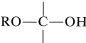formation, like ester formation, depends on formation of the conjugate acid of the carbonyl compound. This is expected to enhance the positive (electrophilic) character of the carbonyl carbon so that the nucleophilic alcohol can add readily to it:The hemiacetal can react further, also with the aid of an acidic catalyst. Addition of a proton can occur in two ways, to give $$7$$ or $$8$$: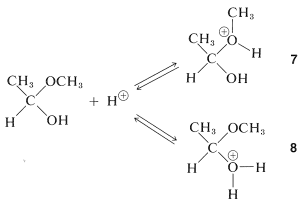The first of these, $$7$$, has $$\ce{CH_3OH}$$ as a leaving group and reverts back to the conjugate acid of ethanal. This is the reverse of acid-catalyzed hemiacetal formation:The second of these,$$8$$, has $$\ce{H_2O}$$ as a leaving group and can form a new entity, the methoxyethyl cation, $$9$$:The ion $$9$$ resemblesand can be expected to behave similarly by adding a second molecule of alcohol to the electrophilic carbon. The product, $$10$$, is then the conjugate acid of the acetal and loses a proton to give the acetal:Formation of hemiacetals and acetals, as well as of hemiketals and ketals, is reversible under acidic conditions, as we already have noted for acid-catalyzed esterification. The reverse reaction is hydrolysis and the equilibrium for this reaction can be made favorable by having an excess of water present: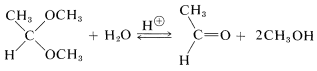The position of equilibrium in acetal and hemiacetal formation is rather sensitive to steric hindrance. Large groups in either the aldehyde or the alcohol tend to make the reaction less favorable. Table 15-3 shows some typical conversions in acetal formation when 1 mole of aldehyde is allowed to come to equilibrium with 5 moles of alcohol. For ketones, the equilibria are still less favorable than for aldehydes, and to obtain reasonable conversion the water must be removed as it is formed.

Table 15-3: Conversion of Aldehydes to Acetals with Various Alcohols (1 Mole of Aldehyde to 5 Moles of Alcohol)
Percent Conversion to Acetal
Aldehyde Ethanol Cycloehexane 2-Propoanol tert-Butyl Alcohol
$$CH_3CHO$$ 78% 56% 43% 23%
$$(CH_3)_2CHCHO$$ 71% - 23% -
$$(CH_3)_3CCHO$$ 56% 16% 11% -
$$C_6H_5CHO$$ 39% 235 13% -